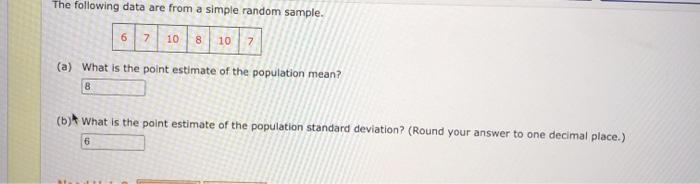### Create an Account

Home / Questions / The following data are from a simple random sample. 6 7 10 8 10 7 (a) What is the point es...

# The following data are from a simple random sample. 6 7 10 8 10 7 (a) What is the point estimate of the population mean? 8 (b) What is the point estimate of the population standard deviation?

The following data are from a simple random sample. 6 7 10 8 10 7 (a) What is the point estimate of the population mean? 8 (b) What is the point estimate of the population standard deviation?Apr 09 2021 View more View LessSubscribe To Get Solution